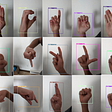# Image Kernels

A picture is worth a thousand words. And technically, A picture is worth a hundred/thousand numbers in the form of matrix within respective range. Let’s validate.

In the above grayscale image, each box/square represent a pixel with the intensity value ranging from 0 to 255. Now, let’s also look at the RGB image.

Within the RGB image, we see there are three values for each pixel which stands for Red, Green, Blue values respectively. Hence, the image is nothing but a matrix of certain dimension.

# Kernel

A kernel is in fact a matrix with an M x N dimension that is smaller than the image matrix. The kernel is also known as the convolution matrix which is well suited for the tasks like blurring, sharpening, edge-detection and similar image processing tasks.

In the below image we have a 5 x 5 grayscale image matrix which is in yellow and the matrix which is in red (3 x 3) is the Kernel matrix to sharpen the overall image. For different image processing tasks the kernel matrix will have varying values.

The kernel matrix will convolve through the big matrix (i.e. image matrix) from left to right and top to bottom, and at each step of convolution, it returns a single pixel value. That single pixel value is the average of the neighborhood pixels in a 3 x 3 matrix grid. Finally, the pixel value returned at each step will result in output image matrix. For example, if we want to sharpen the given image then we can use the defined kernel matrix to achieve that task. and the resultant matrix/image would be a sharpened image. Here is how convolution takes place for a defined region (3 x3).

For a given image select the (x, y) coordinate and align the center of the kernel matrix over the (x, y) coordinate. For a 3 x 3 matrix the center would be 1 x 1. Then multiply each kernel value with the corresponding value of the image matrix and sum all the results of the multiplication. Ideally, it’s the sum of the element-wise multiplication of a matrix. Let’s look at how it works:

The output value (i.e. 241) would be set as pixel value of the output image at (x,y) location. Similarly, the kernel matrix will convolve over the whole image matrix. Now, let’s apply the same kernel on the image and see the difference using OpenCV’s filter2D function.

`import cv2import numpy as npimg = cv2.imread('kernel.png')kernel = np.array([[0, -1, 0],                   [-1, 5, -1],                   [0, -1, 0]])dst = cv2.filter2D(img, -1, kernel)img_concat = cv2.hconcat([img, dst])cv2.imwrite("concat.jpg", img_concat)`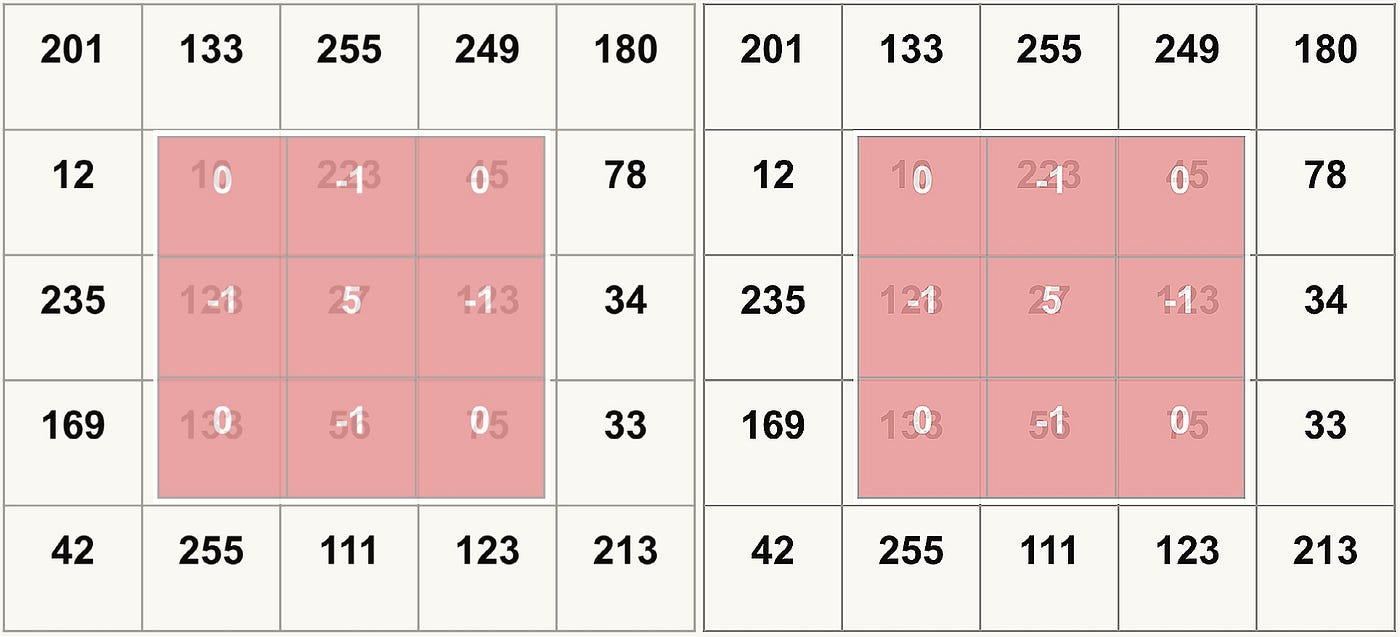(To observe the accurate difference open the image in full screen)

We can observe the difference between the image on the left (i.e. original image) and the image on the right (i.e. sharp image) after applying the given kernel. For operations like blur, edge detection & other image processing tasks the kernel will have different values respectively. Here, we have considered 3 x 3 dimension kernel for simplicity but the kernel can have varying dimensions which is smaller than the image matrix given that the M & N are odd numbers. It’s more of a R&D to come up with the optimal Kernel matrix for a specific task.

To play around with kernel interactively, please refer setosa. Make sure to try out different kernels.

--

--

--

## More from Practical Data Science and Engineering

From Applied Machine Learning to Data Science in the real world; From Modeling to ML Ops; Covering collective experiences reflecting AI, ML and Data Engineering solutions and Operationalising them. Data Engineers and Scientists @Srijan (Srijan Technologies)

## Edit existing FOREIGN KEY constraints in PostgreSQL with the ALTER TABLE command.## Cold Case ch1 pg 14## Get Started with Unity## Finally, I’m Self-Committed to Writing Unit Tests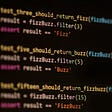## 10 Reasons Why You Should Switch to Linux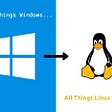## OpenStreetMap is Having a Moment## Week One Documentation## Real Time Object Detection with YOLO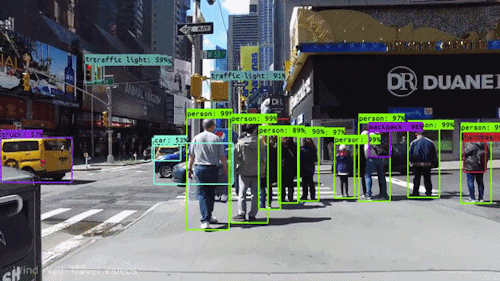## Monitor Social Distancing using Python, YOLOv5, OpenCV## Run Tensorflow 2 Object Detection models with TensorRT on Jetson Xavier using TF C API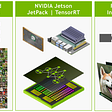## Multi-gesture detection with Tensorflow API ‘TFOD’